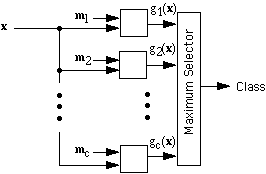Metrics

There is more than one way to define the norm || u ||, and these correspond to different ways of measuring distance, i.e., to different metrics. Two of the most common are
1. Euclidean metric: || u || = sqrt( u12 + u22 + ... + ud2 )
2. Manhattan (or taxicab) metric: || u || = |u1| + |u2| + ... + |ud|
In our template-matching example of classifying characters by counting the number of disagreements, we were implicitly using a Manhattan metric.* For the rest of these notes, we will use either the Euclidean distance or something called the Mahalanobis distance. We will see that
• Contours of constant Euclidean distance are circles (or spheres)
• Contours of constant Manhattan distance are squares (or boxes)
• Contours of constant Mahalanobis distance are ellipses (or ellipsoids)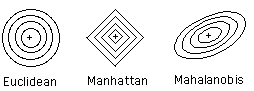Covariance

The covariance of two features measures their tendency to vary together, i.e., to co-vary. Where the variance is the average of the squared deviation of a feature from its mean, the covariance is the average of the products of the deviations of feature values from their means. To be more precise, consider Feature i and Feature j. Let { x(1,i), x(2,i), ... , x(n,i) } be a set of n examples of Feature i, and let { x(1,j), x(2,j), ... , x(n,j) } be a corresponding set of n examples of Feature j. (That is, x(k,i) and x(k,j) are features of the same pattern, Pattern k.) Similarly, let m(i) be the mean of Feature i, and m(j) be the mean of Feature j. Then the covariance of Feature i and Feature j is defined by
c(i,j) = { [ x(1,i) - m(i) ] [ x(1,j) - m(j) ] + ... + [ x(n,i) - m(i) ] [ x(n,j) - m(j) ] } / ( n - 1 ) .

The covariance has several important properties:
• If Feature i and Feature j tend to increase together, then c(i,j) > 0
• If Feature i tends to decrease when Feature j increases, then c(i,j) < 0
• If Feature i and Feature j are independent, then c(i,j) = 0
• | c(i,j) | <= s(i) s(j), where s(i) is the standard deviation of Feature i
• c(i,i) = s(i)2 = v(i)
Thus, the covariance c(i,j) is a number between - s(i) s(j) and + s(i) s(j) that measures the dependence between Feature i and Feature j, with c(i,j) = 0 if there is no dependence. The correspondence between the covariance and the shape of the data cluster is illustrated below.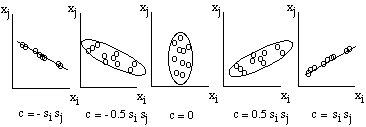Covariance Matrix

All of the covariances c(i,j) can be collected together into a covariance matrix C: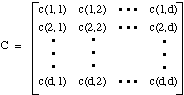This matrix provides us with a way to measure distance that is invariant to linear transformations of the data. Suppose that we start with a d-dimensional feature vector x that has a mean vector mx and a covariance matrix Cx. If we use the d-by-d matrix A to transform x into y through
y = A x ,

it is not hard to show that the mean vector for y is given by
my = A mx,

and the covariance matrix for y is given by
Cy = A Cx A' .

Suppose now that we want to measure the distance from x to mx, or from y to my. We could, of course, use the Euclidean norm, but it would be very unusual if the Euclidean distance from x to mx turned out to be the same as the Euclidean distance from y to my. (Geometrically, that would happen only if A happened to correspond to a rotation or a reflection, which is not very interesting.) What we want to do is to normalize the distance, much like we did when we defined the standardized distance for a single feature. The question is: What is the matrix generalization of the scalar expression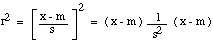The answer turns out to be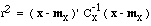.

If you know some linear algebra, you should be able to prove that this expression is invariant to any nonsingular linear transformation. That is, if you substitute y = A x and use the formulas above for my and Cy, you will get the very same numerical value for r, no matter what the matrix A is. Now, suppose there is a feature space in which the clusters are spherical and the Euclidean metric provides the right way to measure the distance from y to my. In that space, the covariance matrix is the identity matrix, and r is exactly the Euclidean distance from y to my. But since we can get to that space from the x space through a linear transformation, and since r is invariant to linear transformation, we can equally well compute r directly from.

Invariants

We can use Invariants to recognise simple objects:
• They provide a simple means of comparison.
• They can provide position and orientation information (i.e. Moments etc.)
• Too simplistic for some applications
• Do not give a unique means of identification.

Invariant Measures

Several of the statistical measures we have met are invariant measures, which is to say that the value of the measure does not vary with, for example, the position of the region in the image, or perhaps its orientation or scale.
Thus, while the centre of gravity of a region obviously varies with its position, its area does not.
While area does vary with scale (and thus closeness of the camera to the object, for example), compactness  as defined above is invariant with respect to scale as well as position and orientation. Invariant measures can be quite useful in recognising objects.
Other invariant measures we have met before are:
• Moments of inertia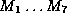are invariant with respect to scaling, rotation and translation.
• Eccentricity is invariant with respect to scaling, rotation and translation.
• Fourier Transforms are rotation invariant. We can use the Fourier transform to compute Fourier descriptors of an object which are invariant with respect to position and orientation.

Linear Discriminants

Recall that when we use a minimum-distance classifier to classify a feature vector x, we measure the distance from x to the templates m1, m2, ..., mc and assign x to the class of the nearest template. Using the inner product to express the Euclidean distance from x to mk, we can write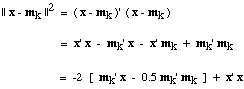Notice that the x'x term is the same for every class, i.e., for every k. To find the template mk that minimizes || x - mk ||, it is sufficient to find the mk that maximizes the bracketed expression, mk' x - 0.5 mk' mk. Let us define the linear discriminant function g(x) by
g(x) = m' x - 0.5 || m ||2 .

Then we can say that a minimum-Euclidean-distance clasifier classifies an input feature vector x by computing c linear discriminant functions g1(x), g2(x), ... , gc(x) and assigning x to the class corresponding to the maximum discriminant function. We can also think of the linear discriminant functions as measuring the correlation between x and mk, with the addition of a correction for the "template energy" represented by || mk ||2. With this correction included, a minimum-Euclidean-distance classifier is equivalent to a maximum-correlation classifier.